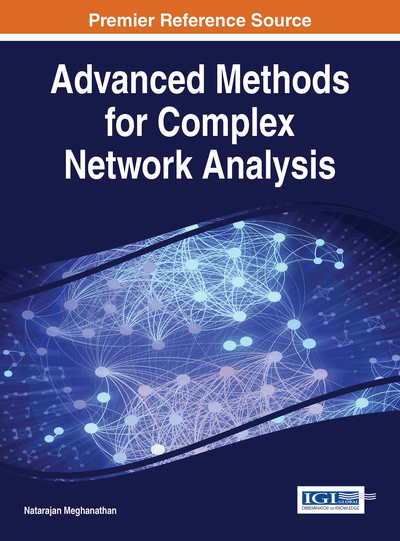Differential Evolution Dynamic Analysis in the Form of Complex Networks

Lenka Skanderova (VSB Technical University of Ostrava, Czech Republic) and Ivan Zelinka (VSB Technical University of Ostrava, Czech Republic)
DOI: 10.4018/978-1-4666-9964-9.ch012
Available
\$37.50
No Current Special Offers

Abstract

In this work, we investigate the dynamics of Differential Evolution (DE) using complex networks. In this pursuit, we would like to clarify the term complex network and analyze its properties briefly. This chapter presents a novel method for analysis of the dynamics of evolutionary algorithms in the form of complex networks. We discuss the analogy between individuals in populations in an arbitrary evolutionary algorithm and vertices of a complex network as well as between edges in a complex network and communication between individuals in a population. We also discuss the dynamics of the analysis.
Chapter Preview
Top

1. Introduction

Differential evolution is a simple yet efficient heuristic originally designed for global optimization over continuous spaces that has been used in many research areas. Thanks to its simple and efficient scheme it is used in many areas of the global optimization research. For example, Kovačević, Mladenović, Petrović, and Milošević (2014) described self-adaptive DE with crossover neighbourhood search for continuous global optimization. Dos Santos Coelho, Ayala, and Mariani (2014) introduced self-adaptive DE based on Gaussian probability distribution, gamma distribution and chaotic sequence. Mlakar, Petelin, Tušar, and Filipič (2015) proposed a novel multi-objective evolutionary algorithm DE for multi-objective optimization based on Gaussian process models.

It is well known, that DE is very sensitive to the choice of the mutation and crossover strategies and their associated control parameters. Tuning of these parameters is time consuming. From this reason, Brest, Greiner, Bošković, Mernik, and Zumer (2006) described DE using self-adaptive control parameters (jDE) where each individual in the population is extended with its own control parameters Fi (mutation constant) and CRi(crossover rate).

Yang, Tang, and Yao (2008) introduced DE with neighbourhood strategy (NSDE) to adapt the mutation constant (scale factor) F. In the same year, these authors presented self-adaptive NSDE (SaNSDE) to improve NSDE's performance where three self-adaptive mechanisms are incorporated: self-adaptation for two candidate mutation strategies (DE/rand/1/bin and DE/best/1/bin), self-adaptations for controlling mutation constant (scale factor) F and crossover rate CR, respectively (Yang, Yao, & He, 2008). Das, Abraham, Chakraborty, and Konar (2009) used a neighbourhood-based mutation operator to improve variants of DE/target-to-best/1/bin scheme.

Mallipeddi and Suganthan (2010) proposed a DE with an ensemble of mutation and crossover strategies and their associated control parameters (EPSDE). EPSDE consists of a pool of mutation and crossover strategies along with a pool of values for each of the associated control parameters. JADE (Iorio & Li, 2005) and DE/current-to-rand/1 (Zaharie, 2009) are used as the mutation strategies and binomial and exponential crossover are used as the crossover strategies.

Islam, Das, Ghosh, Roy, and Suganthan (2012) introduced an adaptive DE algorithm with novel mutation and crossover strategies for global numerical optimization where three algorithmic components have been proposed. First, DE/current-to-gr_best/1 has been introduced, then the modification of the conventional binomial crossover scheme of DE by introducing a fitness-induced bias in the selection of parents from the current generation has been described. Third, the authors suggested the schemes to update the values of the mutation constant F and crossover rate CR in each generation.

DE with composite trial vector generation strategies and control parameters (CODE) was proposed by Wang, Cai, and Zhang (2011) where authors use the strategy candidate pool with three variants of DE, DE/rand/1/bin, DE/rand/2/bin and DE/current-to-rand/1/bin. For each target vector three trial vectors are generated and the best one is then selected to the new generation, if it is better than the target vector.

DE with intersect mutation operator called IMDE was introduced by Zhou, Li, and Gao (2013). In this algorithm, the population of the individuals are divided into two parts – better and worse according to their objective function values. Authors described two novel mutation and crossover operations to generate new individuals. The experimental results showed that the IMDE is better, or at least comparable to above mentioned jDE.

Complete Chapter List

Search this Book:
Reset